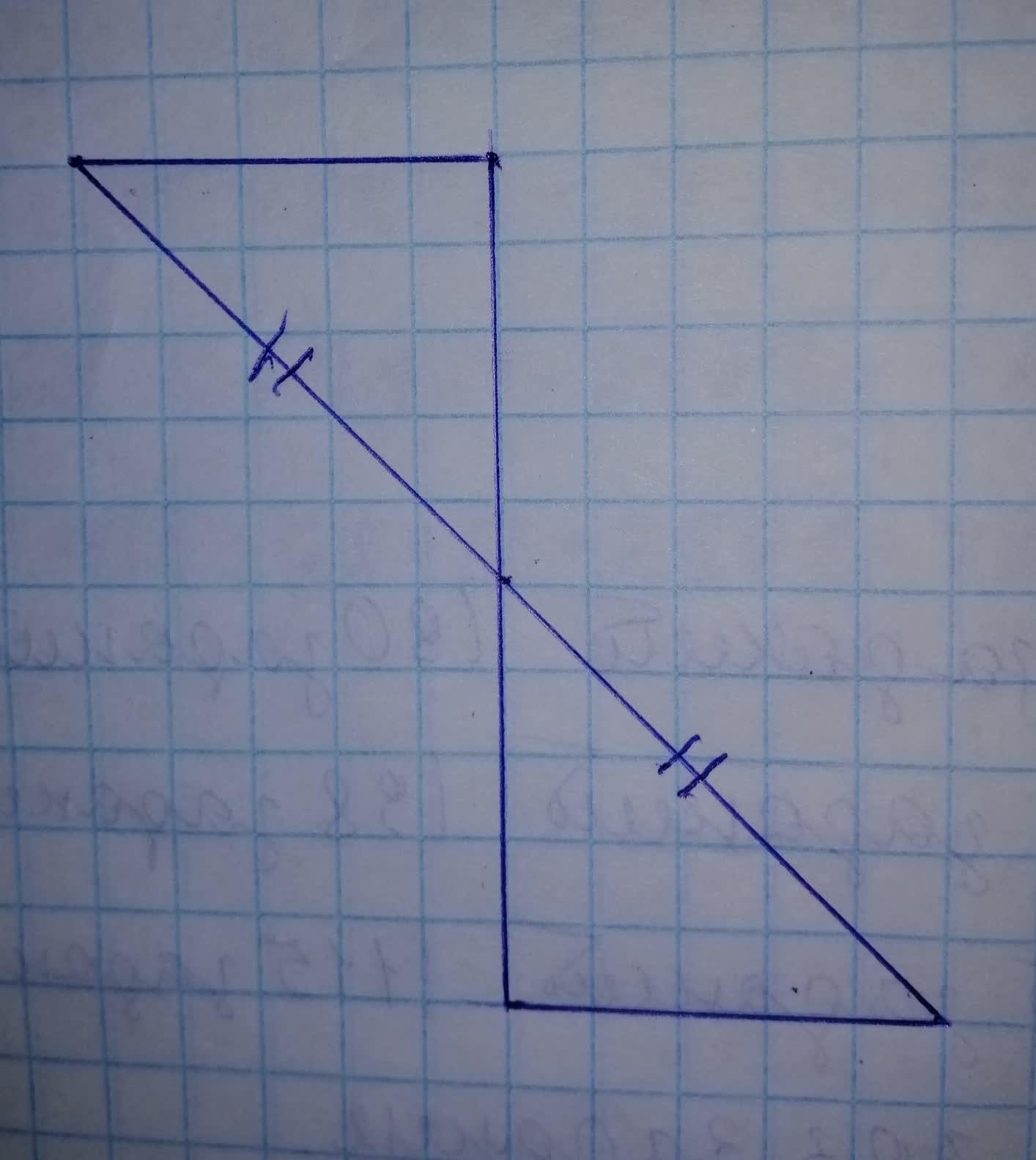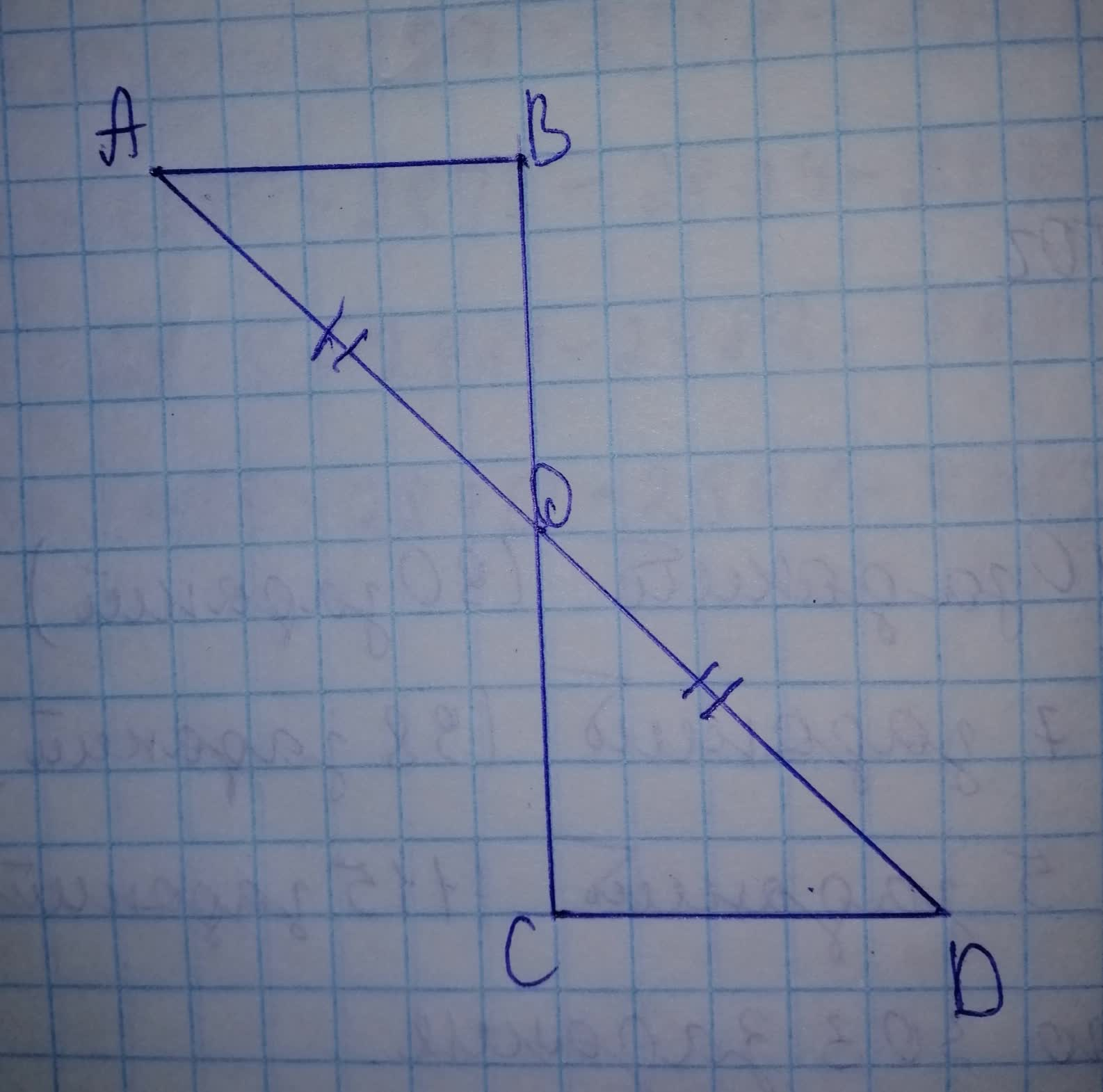tapetivk

2021-11-20

Which statement applies to these triangles: -these triangles must be congruent -these triangles might be congruent -these triangles cannot be congruentStep 1Step 2
In $\mathrm{△}ADB$ and in $\mathrm{△}OCD$
$AO=OD$
$\mathrm{\angle }ADB=\mathrm{\angle }COD$ (vertical opposite angle.)
Can or can not be congruent.

Do you have a similar question?